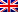#[Cybercamp 2015] [Crypto 11-19] Write Up

## Crypto 11

s=not(t)
s= a xor b
t= b xor c
a=1010

c = ?

This was some easy XOR calculation too :

s xor t = a xor (b xor b) xor c
s xor t = a xor c
s xor t = 1010 xor c
s xor t = c xor 1010

But since s = not(t)
s xor t = 1111

We then have

1111 = c xor 1010

So

#[Cybercamp 2015] [Crypto 1-9] Write Up

Hi guys, since the first crypto challenges were quite easy, I’ll wrap the first of them in this write-up.

## Crypto 1

Ciphered message : DWDFDUIORWDHHXX

This was a really simple Caesar cipher. All letters were shifted 3 times. A became D, W became A and so on.

Deciphered message : ATACARFLOTAEEUU

## Crypto 2

Ciphered message : VCXRKFRQLCDLCKZTFCFI

With a Caesar deciphering with a shift of 17 letters :
Deciphered message : ELGATOAZULMULTICOLOR

## Crypto 3

Ciphered message : BZHUBTLYVZPTWHYLZFXWLTL

First, we found a custom Caesar cipher, with a shift of 7 letters, giving :

USANUMEROSIMPARESYQPEME

Meaning : Use odd numbers YQPEME

I didn’t understand why they talked about odd numbers, because the last word was a progressive Caesar cipher : Q was shifted by 20 letters, then the shift was increased by 2 for each letter.

Deciphered message : USA NUMEROS IMPARES EUREKA

## Crypto 4

Some criminals use a text as a seed for their ciphering keys. Can we find the keys using the following text ? The key words are in bold :

Plantilla: El texto dramático, por lo tanto, es aquel que representa
algún conflicto de la vida a partir del diálogo entre los personajes. La
noción de drama permite nombrar, en forma genérica, a cualquier obra escrita
por un dramaturgo donde los hechos tienen lugar en un espacio y tiempo

By looking at their position in the text, we find :

2 3 5 7 11

These are the first prime numbers. If we keep following this logic, we then have :

2 3 5 7 11 13 17 19 23 29 31 37 41 43 47

And by concatenation of the corresponding words, we have the key :

Deciphered key : textodramaticoloesalgundepartirdialogolaengenericaunhechoslugary

## Crypto 5

Ciphered message : YUc5c1lXMTFibVJ2

After a double base 64 decode, we get the answer

Deciphered message : holamundo

## Crypto 6

a XOR b = 0x35
b XOR C = 0x15

a XOR c = ?

This is some easy XOR calculation.

(a XOR b) XOR (b XOR c) = 0x35 XOR 0x15

a XOR (b XOR b) XOR c = 0x20

a XOR c = 0x20

## Crypto 7

Ciphered message : CHECO VERMO ORAAH

These were some basic anagrams.

Deciphered message : COCHE MOVER AHORA

(Move car now)

## Crypto 9

Find the following row :
RO: 0000 1000 0100 1010 (Initial seed)
R1: 0001 0000 1001 0100
R2: 0100 0010 0101 0000
R3: 0001 0010 1000 00-0
R4: 0101 0000 0100 0010
R5: 0100 0010 0101 0000
R6: ?

We notice that each row is like the first one, but shifted.

R1 is shifted by 2 on the left

R2 is shifted by 3 on the left

then 5, 8. This is Fibonacci. So next line will be shifted by 13

0000 1000 0100 1010

# Introduction

```Session Start: Thu Feb 05 20:49:04 2015 Session Ident: #mastercsi [20:49] * Now talking in #mastercsi [20:49] * Topic is 'http://mastercsi.labri.fr/' [20:49] * Set by admin!~admin on Sat Nov 22 00:06:50 [20:49] and I got an old RSA key that Alice used [20:49] alice, alice's? you gotta be kidding me? [20:49] haha no [20:49] but there was just half, I had to complete with random values to make it work [20:49] it seems to work anyway, if you have something to decipher... [20:49] wait, I have her public key lying around somewhere, and even an encrypted file. I've always wondered what it was ... [20:49] maybe it's the same key? [20:50] I sent you the thing, take a look [21:22] * Disconnected Session Close: Thu Feb 05 21:22:11 2015 ```
The validation key is the message encrypted with the private key of Alice, rebuild it using the following files:

alice.pub
mykey.pem (If you have some issues trying to download this file : right click -> save as …)
secret

# Introduction

```Bob uses a RSA public key with the public exponent e = 65537 and modulus:

N = 55089599753625499150129246679078411260946554356961748980861372828434789664694269460953507615455541204658984798121874916511031276020889949113155608279765385693784204971246654484161179832345357692487854383961212865469152326807704510472371156179457167612793412416133943976901478047318514990960333355366785001217

The challenge validation key is given by the md5sum of the value of its private key d.
```

#[HackingWeek 2015] [Crypto3] Write-up

## Description

Alice wants to use the ECDSA signature algorithm.
For this, she chooses to use the elliptic curve equation E where y2 = x3 – x, defined on the finite field with q elements, Fq, with q = 134747661567386867366256408824228742802669457 .

She chose P as a base point of order N = 2902021510595963727029 with:
P = (18185174461194872234733581786593019886770620, 74952280828346465277451545812645059041440154)

Then, she chooses as secret signature key a random integer d with 1<d<N.
The public key is the point Q=d⊗P where ⊗ is the scalar multiplication on the elliptic curve E.

The value of Q is:
Q=(76468233972358960368422190121977870066985660, 33884872380845276447083435959215308764231090)

The validation key is given by the value of d.